## Frequently Asked Questions: Table Math

With WordPerfect®, the mathematical functionality built into the Table feature is so powerful that most of us have never even considered learning a spreadsheet program. With more than 100 predefined functions, WordPerfect can master almost any mind-boggling number-crunching challenge you can come up with.

Unfortunately, the more powerful the feature, the more you try to do with it C and the more trouble you run into. This article tackles common table math questions and is the second part of a two-part series on frequently-asked table questions. The first article, "Answers to 10 Common Table Formatting Questions", covered common table formatting problems.Q: How do I add a column of numbers?

A: With the QuickSum and Sum functions. The QuickSum function automatically adds the numbers in the cells above (or if the cell above is empty, to the left of) the current cell. It's perfect for columns (or rows) of numbers where you want to place a total in an adjacent cell.

To use QuickSum, click in the cell where you want the total to appear (presumably in the last cell of a row or column of numbers). Then choose either Table > QuickSum, press Ctrl+=, or click the QuickSum button on the toolbar.

The Sum function gives you more flexibility, but it's not quite as fast because you have to build the formula. Sum works on a "range" of cells. A range is a series of cells, so it is shown with the first cell's address and the last cell's address, separated by a colon. A range can be as small as two cells in a column (i.e. A3:A4), as large as 15 rows by 15 columns (i.e. A1:O15), or even larger.

To insert a Sum, position the insertion point in the cell, then choose Table > Sum, or click the Sum button (see Figure 1) on the toolbar. The Sum function determines the correct range by counting the number of cells above it, or, if there aren't any cells above it, by counting the cells to the left.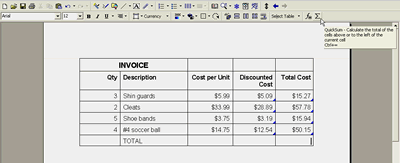Use the QuickSum button on the toolbar to add a column, or row, of numbers in just one click.

If you want to manually adjust the range, you can turn on the Formula toolbar by choosing Table > Formula Toolbar(see Figure 2). Click in the formula text box and make your changes. When you're finished making changes, click the Calculate button to update the totals and then click the Close button.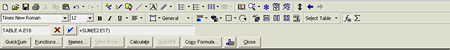The Formula Toolbar gives you access to the tools you set calculations in a table.

Caution: Some cells may contain information that you don't want included in a sum (or any other calculation). You should mark these cells to be ignored when calculating the table. To do so, click in the cell (or select a group of cells) then choose Table > Format > CellIgnore cell when calculating.Q: I can't view the results of a QuickSum. I can see the formula on the Formula toolbar, but nothing shows up in the cell.

A: You may have inadvertently set the numeric format for this cell to Text. Click in the cell where the total should be displayed. ChooseTable > Numeric Format to display the Properties for Table Numeric Format dialog box (see Figure 3). Select your desired format in the Format for numbers in cells section, click Apply, and then click OK. This should reveal the total. If you still don't see a result, delete the contents of the cell and try the QuickSum function again.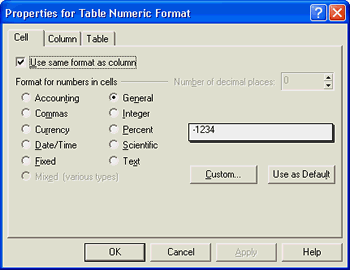Some of the options in the Properties for Table Numeric Format dialog box require you to specify the number of decimal places you want displayed.Q: Why does Sum only add a portion of the column?

A: If you have blank cells or cells that contain text in the middle of the column of calculations, the Sum function won't add the entire column. When you insert a Sum formula in a cell, WordPerfect looks one cell up for a number. If it finds a number, it continues to search upwards until it finds a cell that doesn't contain numbers, then it stops. Therefore, blank cells or cells without numbers cause the calculation to stop.

Furthermore, if a blank cell is found above the total cell, WordPerfect searches to the left to add across the row, so you might be seeing a total for the row, rather than the column.Q: What does the error "Replace Formula?" mean?

A: If you try to delete or edit information that is the result of a formula, WordPerfect stops and displays the Replace Formula message (see Figure 4). This is your opportunity to confirm that you really want to replace the formula, or that you want to bail out because you're about to make a mistake. Be careful here C some formulas are hard to recreate (especially if this isn't your spreadsheet!).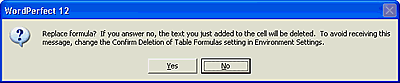The Replace Format message box gives you an opportunity to confirm or reject the replacement of a formula.'

'Q: I'm getting a "circular reference" error message? What does this mean?

A: The "circular reference" message box (see Figure 5) indicates that a formula references its own cell. You can't refer to the formula cell in the formula. For example, if you create the formula "A1+A2" in cell A1, you have created a circular reference. Edit the formula and update the cell reference so that it does not reference the formula's cell.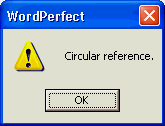The circular reference message box indicates that a formula contains it's own cell addressQ: How can I enter a "constant" into a table formula?

A: One of the great things about WordPerfect tables is that you can create and copy formulas, just like you would in a spreadsheet program. When you copy a formula down a column of numbers, the cell addresses in the formula are automatically adjusted so they reference the correct row number.

For example, if you create a formula of C3*.335, and then copy that formula down through a column of numbers, the cell addresses will be updated automatically to C4*.335, C5*.335, C6*.335, and so on. This "relative addressing" saves you the trouble of having to manually adjust each new formula for the new row number.

There are situations where you don't want a cell address updated. You want to refer to a specific cell, no matter what. To do this, you create an "absolute address" to that cell. Simply surround the cell address with brackets . For example, [C3 C3]*.335 creates an absolute cell address for C3. If you copy this formula down a column of numbers, C3 will not be changed in the formulas.Q: How can I keep a row of formulas on the bottom row if the table is enlarged?

A: Lock the bottom row. This means that the formulas can't be changed and that new rows are inserted above the locked row. So, the bottom row stays put and the Sum function will add up the column, no matter how large it becomes.

To lock the bottom row, select the row, choose Table > Format. Click the Cell tab, then enable the Lock cell to prevent changes check box.Q: Why does WordPerfect run so slow when I'm working in a document with tables?

A: You can set WordPerfect to continuously calculate your table, which is good if you are editing a table, because the calculations are automatically updated. However, in large tables, editing can slow to a crawl as WordPerfect recalculates every formula.

Even if you are editing the text of a document with tables, every time the document is modified, WordPerfect calculates the table to update the totals. This can be maddening, but it's easy to fix. Choose Table > Calculate to display the Calculate dialog box (see Figure 6) and enable the Off option in the Automatic calculation area.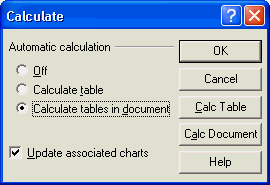The Calculate dialog box lets you turn off automatic calculation in tables.Q: My numbers aren't calculating correctly and I can't figure out why!

A: There could be a number of things wrong (no pun intended). Take a deep breath and work your way through this list. In most cases, you can correct the problem in just a few minutes.

• If column heading cells contain numbers, such as dates, they can be added into the calculation at the bottom of a table. To mark these cells so they won't be included, select the cells, then choose Table > Format. Click the Cell tab and enable theIgnore cell when calculating check box.

• If you have added rows or columns to the table, you should double-check the cell addresses in the formulas.

• Make sure the numbers are not accidentally marked as text. Turn on the formula bar and click in the cell with the number. The number should also appear in the formula bar. If it doesn't, check the Properties for Table Numeric Format dialog box (Table > Numeric Format) and make sure it isn't set to Text. Try changing the number type to Fixed or something other thanGeneral. Also, try deleting and then retyping the number in the cell.

• The math functions require that there be only one number in a cell. Anything after a hard return (text or numbers) is ignored.

• If you are working with large ranges in your Sum formulas, try putting a Sum partway through the numbers to see if that portion is adding correctly. You might be able to determine where the calculations are going wrong so you can just troubleshoot that area.